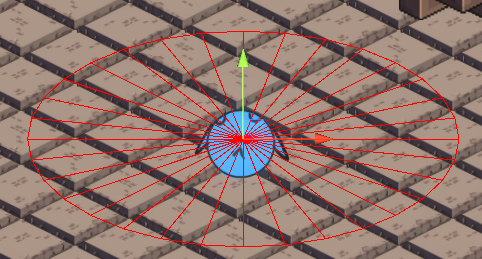# Multiple raycasts around object

I’m trying to detect the player inside an isometric radius. I’m using multiple 2D raycasts hoping to achieve this (I’m open to other solutions), but I just managed to draw the debug lines in the desired pattern. I don’t wanna say I’m dumb, but I tried for hours to shoot the raycasts in this pattern hoping to detect the player, with nor result. Any advice?Code used for drawing the lines:

``````public static void DrawEllipse(Vector3 center, float radius, Color color, int segments, float yDiminisher)
{
Gizmos.color = color;

const float TWO_PI = Mathf.PI * 2;
float step = TWO_PI / segments;
float theta = 0;

float x = radius * Mathf.Cos(theta);
float y = radius * Mathf.Sin(theta);

Vector3 pos = center + new Vector3(x, y, 0);
Vector3 newPos;
Vector3 lastPos = pos;

for (theta = step; theta < TWO_PI; theta += step)
{
y = radius * Mathf.Sin(theta) / yDiminisher;

newPos = center + new Vector3(x, y, 0);

Gizmos.DrawLine(pos, newPos);
Gizmos.DrawLine(center, newPos);

pos = newPos;
}

Gizmos.DrawLine(pos, lastPos);
Gizmos.DrawLine(center, lastPos);
}
``````

Well, I was finally able to di it using a Linecast instead. I’ll leave the code here in case anyone would ever need it

``````public static bool SearchInIsometricRadius(Vector3 center, float radius, int segments, int layerMask)
{
float step = GlobalVars.TWO_PI / segments; // 2 * Mathf.PI / segments

Vector3 newPos;

for (float theta = step; theta < GlobalVars.TWO_PI; theta += step)
{
float x = radius * Mathf.Cos(theta);
float y = radius * Mathf.Sin(theta) / 2;

newPos = center + new Vector3(x, y, 0);

if (Physics2D.Linecast(center, newPos, 1 << layerMask))
return true;
}
return false;
}
``````